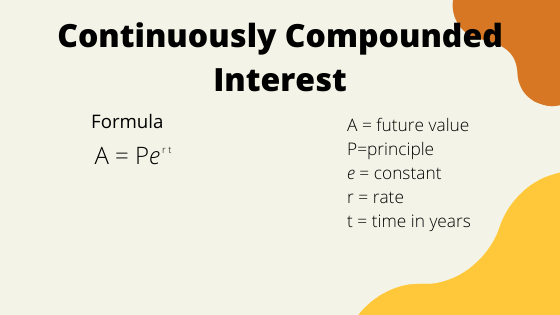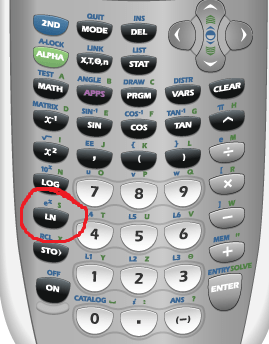# Continuously compounding interest formula with examples

Continuously compounding interest is similar to regular compound interest however, interest is not compounded monthly or quarterly but instead, continuously. The continuously compounding interest formula can be used to find the future value of an investment at a given rate or the amount of time it takes to reach a future value given a desired amount. This is commonly taught in college algebra courses or sometimes calculus courses. Below we will look at the formula and some examples of using it.

## Examples of finding the future value with the continuously compounding interest formula

We will start with using the formula to calculate the future value of an investment. Then we will look at how to find the time it takes to reach a given future value.Remember that $$e$$ is a constant. This is located on your calculator and you should use that instead of an approximation in order to get the most accurate answer.

An investment of $12,000 is invested at a rate of 3.5% compounded continuously. What is its value after 6 years? ### Solution Determine what values are given and what values you need to find. Then, use the formula. • Investment of$12,000 – this is the principle: $$P=12000$$
• Rate 3.5% – remember to write this as a decimal (divide by 100): $$r = 0.035$$
• Value after 6 years: $$t = 6$$

You are finding the value 6 years in the future, so you are finding $$A$$, the future value.

\begin{align}A &= Pe^{rt}\\ &= 12000e^{0.035\times6}\\ &= \bbox[border: 1px solid black; padding: 2px]{14,804.14}\end{align}

Answer: The value will be $14,804.14 after 6 years. On your calculator, if you are using a TI83 or TI 84, you should have the following.The $$e$$ is located just above $$LN$$.## Example of finding the time to reach a certain value using the continuously compounding interest formula In some problems, you may be given a goal value such as$10,000 and asked how long it will take to reach that value given an initial investment. Here, you will use the fact that $$\ln(x)$$ is the inverse of $$e^x$$.

### Example

An investment of $5,500 is made at a continuously compounding rate of 2%. How many years will it take to reach a value of$15,000?

### Solution

Again, review the values you know and what you are trying to find.

• Investment of $5,500 so $$P = 5500$$ • Rate 2% so $$r = 0.02$$ • Reach a value of$15,000 – this is the future value so $$A = 15000$$

We are asked the time, $$t$$. Now use the formula. Recall the statement above that $$\ln(x)$$ is the inverse of $$e^x$$.

\begin{align}A &= Pe^{rt}\\ 15000 &= 5500e^{0.02\times t}\\ \frac{15000}{5500} &= e^{0.02\times t}\\ \frac{30}{11} &= e^{0.02\times t}\\ \ln\left(\frac{30}{11}\right) &= \ln\left(e^{0.02\times t}\right)\\ \ln\left(\frac{30}{11}\right) &= 0.02t\\ \frac{\ln\left(\frac{30}{11}\right)}{0.02} &= t \\ \bbox[border: 1px solid black; padding: 2px]{50.17} &= t \end{align}